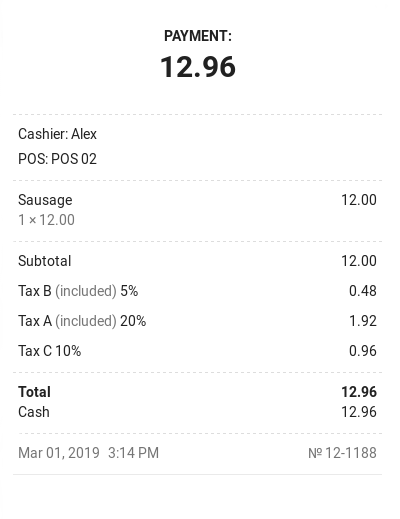# How Taxes are Calculated

There are two types of taxes that can be applied to items at Loyverse POS (Play Market or App Store) : ‘added to the price’ and ‘included in the price’.
An example of ‘added to the price’ tax is sales tax in the USA - where all the prices are listed without the sales tax, but during checkout, the sales tax is added to the price, and the customer pays the price of the item plus the sales tax.
An example of ‘included in the price’ tax is value-added tax (VAT) in EU countries, also known as goods and services tax (GST) in India, Australia, Canada, Malaysia, New Zealand, and Singapore - where the tax is already included in the price listed.

## Calculation of ‘added to the price’ tax

To calculate the value of the ‘added to the price’ tax, you have to multiply the item price by the tax rate in decimal form.
For example, if the tax rate is 6%, multiply the item’s price by 0.06. So if the item is $20, the value of the ‘added to the price’ tax will be 20×0.06=$1.20.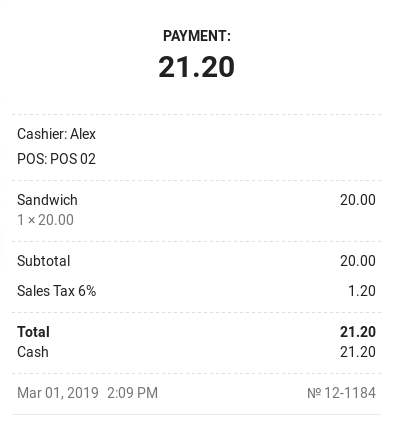## Calculation of ‘included in the price’ tax

In the case of ‘included in the price’ tax, the item price consists of pre-tax price value plus the tax value.
Price = PretaxPrice + Tax
And the tax will be applied to the pre-tax price.
To calculate the item's pre-tax price, divide the price by 1 + the tax rate in decimal form. To find the value of the tax that is included in the item’s price, you have to subtract the pre-tax price from the item price.
For example, if the tax rate is 6%, divide the item’s price by 1.06. So if the item is $20 with the ‘included in the price’ tax, then the value of the pre-tax price is 20/1.06 =$18.87 (rounded), and the value of the ‘included in the price’ tax is 20-18.87=$1.13.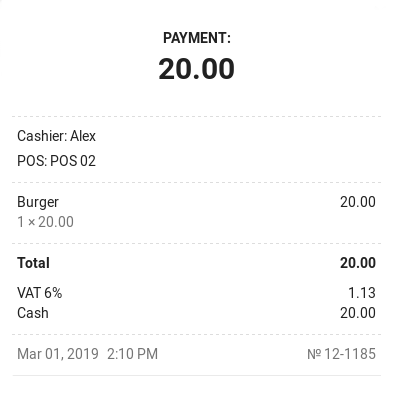### Application of several taxes at the same time How to calculate the tax value if multiple ‘added to the price’ taxes were applied to an item The value of multiple ‘added to the price’ taxes of an item is calculated in the same way as if only one tax is applied (see above). For example, if you sell an item at$12.00 with added tax A=20% and added tax B=5%, the tax values will be $2.40 and$0.60 respectively:
TA = 12×0.20=2.4 ; TB = 12×0.05=0.6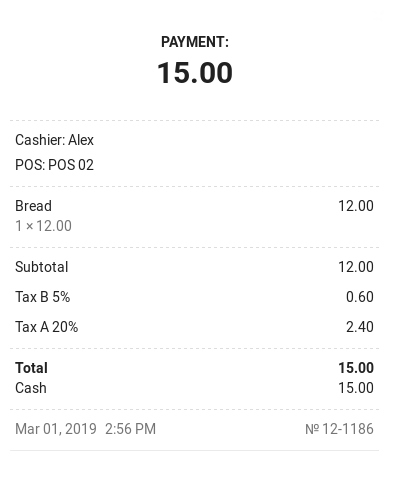How to calculate the tax value if multiple ‘included in the price’ taxes were applied to an item
The value of ‘included in the price’ tax of an item is calculated as the multiplication of the price of the item and the tax rate, divided by the sum of the rates of all included taxes plus one:

$$TI = {p × TIPV \over (1+\sum\nolimits_{i} TIPV_{i})}$$

where
TI = the value of the ‘included in the price’ tax
p =  the price of the item
TIPV = the included tax rate in decimal form
i = the number of tax rates included in the price

For example, if you sell an item at $12.00 with included tax A=20% and included tax B=5%, the value of TI will be$1.92 and $0.48 respectively: $$TI_{A} = {12 × 0.2 \over (1+0.2+0.05)}=1.92$$ $$TI_{B} = {12 × 0.05 \over (1+0.2+0.05)}=0.48$$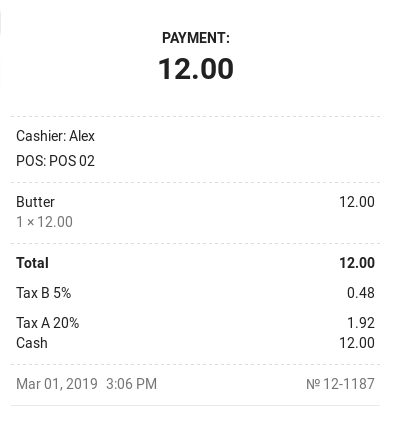How to calculate the value of the ‘added to the price’ tax if the price has included taxes The value of the ‘added to the price’ tax of an item is calculated as the multiplication of the rate of the added tax by the price of the goods reduced by the sum amount of the included taxes: $$TA = {TAPV × (p -\sum\nolimits_{i} TI_{i})}$$ where TA = the value of the ‘added to the price’ tax p = the price of the item TAPV = the added tax rate in decimal form (1%=0.01) TI = the value of the ‘included in the price’ tax i = the number of tax rates included in the price For example, if you sell an item at$12.00 with included tax A=20% and included tax B=5%, and also added tax C=10%, the value of TA will be \$0.96:

$$TA = {0.1 × (12 -(1.92+0.48)) = 0.96}$$